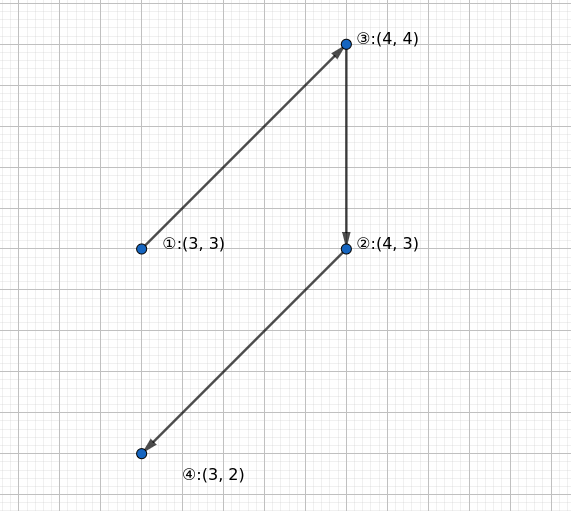# #604. 【UER #9】赶路

### 样例一

#### input

1
4
3 3
4 3
4 4
3 2



#### output

1 3 2 4



#### explanation### 限制与约定

$1$ $10$
$2$
$3$ $15$
$4$
$5$ $500$ $x_1 = y_1 = 0$ 且四个象限均存在点
$6$ $x_1 \leq x_i \leq x_n$
$7$
$8$
$9$
$10$